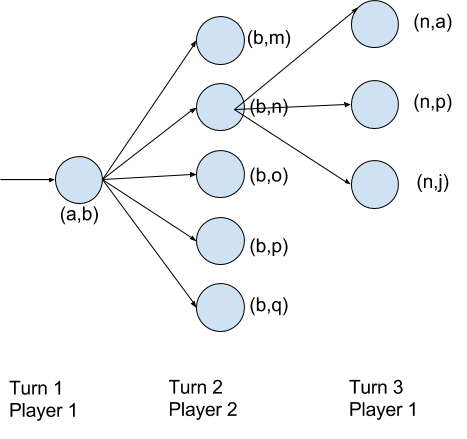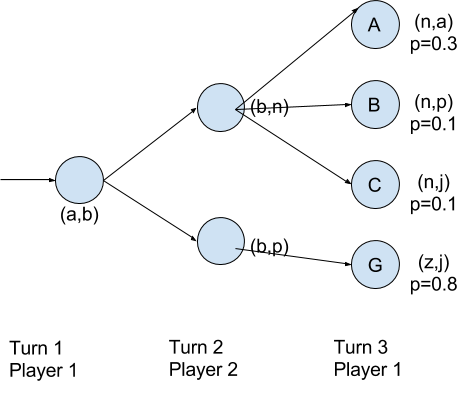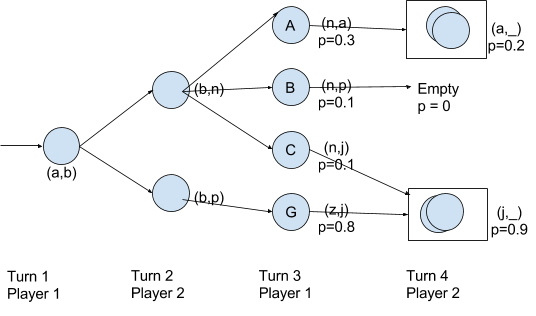For those blissfully unaware, Antakshari is a singing game played by two or more teams. The starting team sings the first verse of a song, and the next team has to pick a song starting with the last letter of the previous team’s song. Songs cannot be repeated in the game. For instance, if the first team sings a song starting with म ‘Ma’ which ends with न ‘Na’, the next team has to sing a song starting with न ‘Na’. Subsequently, none of these songs can be repeated. Scoring is ambiguous and varies during casual play, but we can assume that once a team is unable to pick a song within a reasonable amount of time, they lose a point. Usually, before this state is reached, humans tend to have several arguments about whether a song was already done, and move on to other games.

## Expressing the problem

For the sake of this article, I will limit the game to two players. I’ll also consider English characters, though technically, any set of symbols can be used. Trying to express the game more formally:

Each song can be represented as a tuple with two symbols representing the starting and ending symbol. For example: (a,b) represents a song starting with ‘a’ and ending with ‘b’.

Now let’s define a bunch of sets: S: Set of all songs Sₓ: Set of all songs starting with a symbol x K: Set of songs which have been exhausted (or killed, by singing them very poorly. Thus, ‘K’ for killed). When the game starts, K will be empty, and an element will be added after every turn.

For every turn `t = (a,b)`, the next valid turn can be defined as some u, such that `u ∈ Sb ∩ K'` (Meaning, the tuple u belongs to the set of songs starting with b, but not in K). Two distinct songs can be represented with the same tuple, but they won’t be equal (so the Set representation may not be intuitive to work with).

We can also represent the set of possible moves as a graph, like so:## Strategizing

Let’s say that two players are playing to win, how can we determine the best possible move? Or can we determine what would constitute as a good move or a bad move?

One possible option would be to minimize the number of choices you give your opponent. In the graph above, let’s say we are at Turn 2 for Player 2. We have 5 options to pick from {(b,m), (b,n), (b,o), (b,p), (b,q)}. We can see that (b,n) will give Player 1 three choices in the next turn, and the rest are omitted from the diagram.

If one of the options, say (b,q) has zero choices, then we can simply pick that one and win, since Player 1 won’t have any moves left. On the other hand, if we don’t have a node with zero choices, we can simply pick the one which gives the least number of choices to the opponent.

But I don’t like this strategy because in a real game, things won’t work simply by minimizing the number of choices the opponent has. To elaborate, let’s say you have a choice between node A which has 3 popular songs vs. node B which has 5 obscure songs, the better choice would be node B, since your opponent would be less likely to remember the obscure songs.

Now let’s try to represent the obscurity (or popularity) of a song. We can simply say that it is the probability of a song being “known” and we will represent it with some p where 0 ≤ p ≤ 1. (A song with p=1 will be definitely known, and one with p=0 will be definitely unknown).

A song being “known” is a bit ambiguous, but let’s say we can come up with this value using a clever algorithm that looks at music charts, top-ten lists, revenue generated by the song, and so on. Heck, let’s even throw in the profile of your opponent in there. (e.g. if they’re really into a particular genre or artist, they may know the more obscure ones in that category as well). In the end, you have some value p which represents the likelihood of a particular song being remembered by your opponent.

Let’s re-look at a graph of moves, this time with probabilities added.I’ve limited the number of nodes for the sake of brevity. Now let’s say we are at Turn 2 Player 2, we need to pick the next possible move between (b,n) and (b,p) (or any of the other potential options we have). In this case, we’d pick the one that has the total lowest probability of being known.

We can claim that the knowledge of songs is independent, but not mutually exclusive. (Typically, your knowledge of song A won’t affect your knowledge of song B (=> independent). Also, knowing song A and knowing song B are not like a coin-toss where only one of the two can occur (=> not mutually exclusive)).

So then, from the graph we can calculate the probability of knowing A or B or C as: P ( A ∪ B ∪ C) = P(A) + P(B) + P(C) - P(A∩B) - P(B∩C) - P(A∩C) + P(A∩B∩C) = 0.433 The formula can be extended for any number of nodes. (Although it would get ugly, and there’s probably a simplification available).

The other choice P(G) has a popularity of 0.8, which is greater and 0.433. So the better choice for Player 2 is to go with (b,n) even though it has more number of options for your opponent.

### Looking at choices you’ll get back

To further complicate the problem, let’s say you are Player 2 at Turn 2, and instead of only looking at Turn 3, you also want to look at Turn 4, where it will be your turn to play again. Why would you want to do that? To ensure that you get back a set of choices that have a high probability of being known by yourself. That is, you shouldn’t drive yourself into a trap.If Player 2 had chosen (b,n), (since it had the lower popularity score), then at Turn 3, Player 1 would most likely choose Node A (n,a) and Player 2 would find themselves in a soup, because the next set of choices have a combined popularity of only 0.2. On the other hand, if Player 1 chose node C, (even though that would be less likely), Player 2 would have no problem playing Turn 4, since the combined popularity is 0.9. Or if Player 1 chose (n,p), then Player 2 would lose!

It would make sense to add a safety heuristic to our algorithm. We could pick a safe popularity value such as 0.8 (or how much ever a player is willing to bet), and at no point should we pick a choice if the subsequent move would end up below our safety threshold.

Looking ahead will have diminishing returns. It would not make sense to compute all possible choices and look ahead more than a few turns. We can use conditional probability to calculate which branches of the graph to look into. For instance if Player 2 has a choice X and in the next turn, Player 1 ends up with A and B as possible choices subsequently, we can compare `P(A|X)` and `P(B|X)` and disregard the branch that have a low chance of occurring, again by defining some threshold.

## Practical Considerations

While the formulations above make sense mathematically, they have very little practical applicability in my opinion.

• If a human player is playing against a machine, the human is already at a disadvantage because she would have limited memory of songs, while the computer can look it up in fractions of a second, regardless of the strategy it employs. The computer would simply have a larger database.
• If two computers are playing against each other, then the popularity score makes no sense - all the songs would be in their memory.
• If two humans were playing, and one of them cheating by using a computer, then they wouldn’t be able to sing a song that the computer suggests if they didn’t know it. If both humans were cheating, it would be equivalent to two computers playing against each other, with the added confusion of the computers suggesting obscure songs that the humans aren’t aware of.

Nonetheless, if we keep the strategizing part aside, we can still answer some interesting questions like: What would be the longest and shortest possible Antakshari game?

And from a computational perspective, Antakshari turned out to be more complex than I had anticipated, more so if you consider strategizing. Building the graph of next possible moves in an optimal way would itself be an interesting challenge, albeit perhaps a solved one, since it does resemble other board games.

## Conclusion

This was a fun little exercise I partially came up with while other humans were playing the actual game. If you have thoughts, comments, or if you notice any errors, do leave a comment.